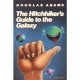# drawTriangles() Cubes

Actionscript:
1. private function addCube(xp:Number, yp:Number, zp:Number, w:Number, h:Number, leng:Number):void{
2.             var hw:Number = w * 0.5;
3.             var hh:Number = h * 0.5;
4.             var hl:Number = leng * 0.5;
5.             var xA:Number = xp - hw;
6.             var xB:Number = hw + xp;
7.             var yA:Number = yp - hh;
8.             var yB:Number = hh + yp;
9.             var zA:Number = zp - hl;
10.             var zB:Number = hl + zp;
11.             _verts.push(xA, yA, zA,
12.                         xB, yA, zA,
13.                         xA, yB, zA,
14.                         xB, yB, zA,
15.                         xA, yA, zB,
16.                         xB, yA, zB,
17.                         xA, yB, zB,
18.                         xB, yB, zB);
19.
20.             var index:int = _boxIndex * 8;
21.             var i0:int = index, i1:int = index + 1, i2:int = index + 2;
22.             var i3:int = index + 3,  i4:int = index + 4, i5:int = index + 5;
23.             var i6:int = index + 6, i7:int = index + 7;
24.             _indices.push(i0, i1, i2,
25.                           i1, i3, i2,
26.                           i6, i7, i4,
27.                           i7, i5, i4,
28.                           i1, i5, i3,
29.                           i7, i3, i5,
30.                           i4, i5, i0,
31.                           i1, i0, i5,
32.                           i2, i6, i0,
33.                           i0, i6, i4,
34.                           i2, i3, i6,
35.                           i3, i7, i6);
36.
37.             _faces.push(new Face(), new Face(), new Face(),
38.                         new Face(),  new Face(), new Face(),
39.                         new Face(), new Face(), new Face(),
40.                         new Face(), new Face(), new Face());
41.             _uvts.push(Math.random(), Math.random(), 0,
42.                        Math.random(), Math.random(), 0,
43.                        Math.random(), Math.random(), 0,
44.                        Math.random(), Math.random(), 0,
45.                        Math.random(), Math.random(), 0,
46.                        Math.random(), Math.random(), 0,
47.                        Math.random(), Math.random(), 0,
48.                        Math.random(), Math.random(), 0);
49.             _boxIndex++;
50.         }

Lately I've been posting large code snippets... so today I'm highlighting part of a larger snippet - The above code is the heart of a small experiment I created this morning. It sets up a cube for use with drawTraingles().

The rest of the code can be read here:
Cubes3D.as

Have a look at the swf here...

1.Jackson Dunstan
Posted September 26, 2009 at 4:03 pm | Permalink

This is really cool and a much more efficient way than the major Flash 3D engines if all you’re concerned about is cubes. Thanks for the nice writeup and demo app!

2.Zevan
Posted September 30, 2009 at 9:18 am | Permalink

thanks jackson, glad you like it…

3.Riccardo
Posted November 13, 2009 at 8:28 am | Permalink

Really great examples, thank you!

I noticed just a problem: the object’s 3D positions are very different if you publish the swf in a html page or if you open the swf as a standalone. Maybe a relative coordinates problem?

4.Riccardo
Posted November 23, 2009 at 8:45 am | Permalink

I found sometimes the same problem even playing the swf in a browser. It’s not systematic, it can be happen in every browser/OS. Any ideas?5.chris aivazidis
Posted September 16, 2010 at 4:22 am | Permalink

Very rare stuff! Congrats! With your permission I like to use it for a fancy menu in one of my personal web pages. Should be ok with u (new in As3 myself) how can I attach a label in to each side of each box? (textfield or movie clip). Your help will be greatly appreciated.

6.Zevan